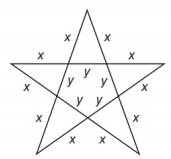Chapter A.1, Problem 27EElementary Geometry For College St...

7th Edition
Alexander + 2 others
ISBN: 9781337614085

Solutions

Chapter
SectionElementary Geometry For College St...

7th Edition
Alexander + 2 others
ISBN: 9781337614085
Textbook Problem

A large star is to be constructed, with lengths as shown in the accompanying figure. Give an expression for the total length of the wood strips used in the construction.To determine

To find:

The expression for the total length of the wood strips which are used for the construction of a large star.

Explanation

1) In FOIL method, First, Outside, Inside, and Last pairs of terms are multiplied and then added.

2) The area of the rectangle is given by LW where L is the length and W is width of the rectangle.

Calculation:

There are 10 strips of length x and 5 strips of length y in the given large star...

Still sussing out bartleby?

Check out a sample textbook solution.

See a sample solution

The Solution to Your Study Problems

Bartleby provides explanations to thousands of textbook problems written by our experts, many with advanced degrees!

Get Started

Subtract: 60,0004,803

Elementary Technical Mathematics

In Exercises 7-10, find the slope of the line shown in each figure. 7.

Applied Calculus for the Managerial, Life, and Social Sciences: A Brief Approach

In Problems 35-38, use the x-intercept method to find one solution of each equation.

Mathematical Applications for the Management, Life, and Social Sciences

Find f(x) if f(x) = 10x2 + cos x. a) 20x sin x + C b) 20x cos x + C c) 103x3cosx+C d) 103x3+sinx+C

Study Guide for Stewart's Single Variable Calculus: Early Transcendentals, 8th

True or False: The function f is continuous at (0, 0), where

Study Guide for Stewart's Multivariable Calculus, 8th